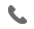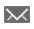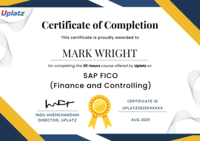•+44 7459 302492info@uplatz.com
• Register

# Numerical Computing in Python with NumPy

Master Numerical Computing and Data Manipulation with NumPy: Python's essential Data Science Library. Apply NumPy for data science & machine learning.
##### Preview Numerical Computing in Python with NumPy course
Price Match Guarantee   Full Lifetime Access     Access on any Device   Technical Support    Secure Checkout   Course Completion Certificate
•88% Started a new career BUY THIS COURSE (GBP 29)
•93% Got a pay increase and promotion
Bestseller
Trending
Job-oriented
Instant access

#### Students also bought -

Completed the course? Request here for Certificate. ALL COURSESNumPy, short for "Numerical Python," is a powerful library in Python designed for numerical computations and data manipulation. It forms the foundation of many data science, scientific, and engineering applications in Python.

NumPy introduces the ndarray, a multi-dimensional array object that allows efficient storage and operations on large datasets. These arrays can be one-dimensional (vectors), two-dimensional (matrices), or even higher-dimensional. NumPy provides a vast collection of mathematical functions and routines that work seamlessly with arrays, enabling vectorized operations and speeding up computations compared to traditional loop-based approaches.

Additionally, NumPy supports broadcasting, a feature that automatically extends the shapes of smaller arrays to perform element-wise operations with larger arrays. This makes it possible to perform operations on arrays with different dimensions, simplifying code and increasing efficiency. NumPy's integration with other libraries, such as SciPy for scientific computing and Matplotlib for data visualization, creates a powerful ecosystem for data analysis and numerical computation in Python. Its widespread adoption and ease of use have made NumPy an essential tool for data scientists, researchers, engineers, and anyone involved in numerical computing tasks.

NumPy offers several features that make it incredibly useful in data science and machine learning applications:

1. N-dimensional Arrays: NumPy provides the ndarray data structure, enabling the creation and manipulation of multi-dimensional arrays. These arrays can efficiently store and process large datasets, making it easier to work with complex data structures common in data science.

2. Broadcasting: NumPy supports broadcasting, which allows element-wise operations on arrays with different shapes and dimensions. This simplifies calculations and makes code concise, enhancing performance.

3. Vectorized Operations: NumPy allows vectorized operations, where mathematical operations are applied element-wise to entire arrays. This is significantly faster than traditional loop-based operations and enhances performance.

4. Mathematical Functions: NumPy provides an extensive library of mathematical functions and routines for array computations. It includes functions for basic arithmetic, trigonometry, logarithms, statistics, linear algebra, and more.

5. Integration with other Libraries: NumPy integrates seamlessly with various Python libraries used in data science and machine learning, such as SciPy (for scientific computing), pandas (for data manipulation), and Matplotlib (for data visualization). This interoperability creates a powerful ecosystem for data analysis and exploration.

6. Efficient Memory Usage: NumPy uses contiguous memory blocks, leading to efficient memory management and reduced overhead during data operations.

7. Random Number Generation: NumPy includes facilities for random number generation, essential for simulating datasets and performing statistical analyses.

In data science and machine learning, NumPy's features play a crucial role:

1. Data Preparation: NumPy's multi-dimensional arrays are used to store and manipulate datasets, making it easier to preprocess data, perform filtering, and apply feature engineering techniques.

2. Mathematical Operations: NumPy's mathematical functions enable vectorized calculations, making complex numerical computations more efficient. This is especially beneficial when dealing with large datasets in machine learning algorithms.

3. Linear Algebra: NumPy supports linear algebra operations, such as matrix multiplication and solving systems of equations, which are fundamental to machine learning models like linear regression and neural networks.

4. Interoperability: As a fundamental library, NumPy is used alongside other data science libraries like pandas and SciPy, allowing seamless data transformations, analysis, and visualization.

In summary, NumPy's powerful array operations, efficient memory usage, and seamless integration with other libraries make it an indispensable tool for data scientists and machine learning practitioners. Its widespread adoption in the Python data science ecosystem underscores its critical role in enabling high-performance numerical computing tasks.

### Course/Topic - Python Programming (advanced) - all lectures

• In this lecture session of python programming we learn about the basic introduction of Numpy and also talk about features of Numpy in python programming.

• 22:20
• In this lecture session we learn about Numpy tutorial basics and also talk about functions and importance of Numpy in python programming in advance.

• 17:05
• In this lecture session we learn about Numpy attributes and functions in python programming in python programming and also talk about features of Num py attributes.

• 24:43
• In this lecture session we learn about creating arrays from existing data and also talk about how we create arrays in the best way and features of array.

• 24:51
• In this lecture session we learn about creating arrays from ranges in python programming and also talk about features of creating arrays from ranges in brief.

• 28:43
• In this lecture session we learn about indexing and slicing in Numpy and also talk about features and importance of indexing and slicing in Num py.

• 15:39
• In this lecture session we learn about advanced slicing in Num py and also talk about features of advanced slicing in Numpy.

• 29:55
• In this lecture session we learn about append and resize functions in python programming and also talk about functions of append and resize functions.

• 25:20
• In this lecture session we learn about Nditer function and broadcasting in python programming and also talk about Nditer functions.

• 24:23
• In this lecture session we learn about Nditer functions in python programming and also talk about features of Nditer functions in brief.

• 26:17

• 10:42

• 07:40
• In this lecture session we learn about Numpy broadcasting functions in python programming and also talk about functions of Num py broadcasting.

• 07:12
• In this lecture session we learn about arrays manipulation functions in python programming and also talk about features of array manipulation functions.

• 29:18
• In this lecture session we learn about Num py unique in python programming and also talk about features of Num py uniques.

• 16:52
• In this lecture session we learn about Numpy delete in python programming and also talk about features of Numpy delete().

• 10:24
• In this lecture session we learn about containing alternate values from array deleted and also talk about double dimension arrays.

• 05:45
• In this lecture session we learn about Num py insert function in python programming and also talk about different types of function in python.

• 10:22
• In this lecture session we learn about Num py Ravel() swapaxes in python programming and also talk about features of swapaxes().

• 14:43
• In this lecture session we learn about split function in python programming and also talk about some functions and features of split function.

• 11:53
• In this lecture session we learn about HSplit functions in python programming and also talk about the importance of HSplit functions.

• 12:18
• In this lecture session we learn about left shift and right shift functions in python programming and also talk about features of left shift and right shift functions.

• 07:09
• In this lecture session we learn about left shift and right shift functions in python programming and also talk about features of left shift and right shift functions.

• 11:44
• In this lecture session we learn about In this lecture session we learn about and also talk about features of NumPy Trigonometric Functions.

• 14:40
• In this lecture session we learn about Numpy round functions in python programming and also talk about factors of round functions.

• 14:16
• In this lecture session we learn about Num py arithmetic functions in python programming and also talk about features of arithmetic functions.

• 07:43
• In this lecture session we learn about Num py power and reciprocal functions in python programming and also talk about features of Num py power and reciprocal functions.

• 07:51
• In this lecture session we learn about Num py power and mod function in python programming and also talk about features of mod functions.

• 06:36
• In this lecture session we learn about Num py Imag() and real() in python programming and also talk about features of Imag() real() functions.

• 08:06
• In this lecture session we learn about Numpy concatenate functions in python programming and also talk about factors and features of concatenate functions.

• 07:50
• In this lecture session we learn about Num py statistical functions in python programming and also talk about features of statistical functions.

• 06:22
• In this lecture session we learn about Numpy statistical functions in python programming and also talk about mean median Ptp functions in brief.

• 22:42
• In this lecture session we learn about Num py average functions in python programming and also talk about features of average functions.

• 21:25
• In this lecture session we learn about Num py sort search counting and also talk about factors of search counting functions in brief.

• 20:09
• In this lecture session we learn about Num py search sort algorithms in python programming and also talk about features of search sort algorithms in brief.

• 06:54
• In this lecture session we learn about Sort() functions and also talk about features of Sort() function in python programming.

• 06:10
• In this lecture session we learn about Numpy sort functions and also talk about features Sort function in brief.

• 16:40
• In this lecture session we learn about Numpy argsort in python programming and also talk about features of argsort in brief.

• 07:12
• In this lecture session we learn about Non zero where in python programming and also talk about function of Nonzero where in brief.

• 14:23
• In this lecture session we learn about Extract and Int in python programming and also talk about key features of Extract.

• 06:32
• In this lecture session we learn about argmax() and argmin in python programming and also talk about features of argos and argmin.

• 07:34
• In this lecture session we learn about Byteswap copies and views in python programming and also talk about features of Byteswap couples and views.

• 25:00
• In this lecture session we learn about str functions in Numpy in python programming and also talk about features of str functions.

• 13:32
• In this lecture session we learn about string function in numpy add and also talk about features of ADD() and Multiply() functions.

• 05:59
• In this lecture session we learn about Numpy centers in python programming and also talk about functions of Numpy centers.

• 08:18
• In this lecture session we learn about capitalize centers in python programming and also talk about features of capitalize centers.

• 12:16
• In this lecture session we learn about string functions in python programming and also talk about features of string functions.

• 17:36
• In this lecture session we learn about String functions in advance and string functions in python programming in brief.

• 08:27
• In this lecture session we learn about Numpy matrix library in python programming and also talk about features of Numpy matrix in brief.

• 18:25
• In this lecture session we learn about Numpy joining arrays in python programming and also talk about functions of arrays joining.

• 21:01
• In this lecture session we learn about Linear algebra in python programming and also talk about features of linear algebra.

• 13:55
• In this lecture session we learn about features of Linear algebra in python programming and also talk about the importance of linear algebra.

• 13:39
• In this lecture session we learn about Linear algebra examples and also talk about real time examples of linear algebra.

• 11:20
• In this lecture session we learn about Linear algebra features and real time examples of Arrays functions using linear algebra.

• 06:46
• In this lecture session we learn about the program of determination in matrix using linear algebra and also talk about features of determination in matrix.

• 08:40
• In this lecture session we learn about inverse of matrix using linear algebra and also talk about features of inverse of algebra.

• 16:44
• In this lecture session we learn about the program of inverse of matrix in brief and also talk about functions of inverse of matrix.

• 16:32
• In this lecture session we learn about random modules in python programming and also talk about features of random modules in brief.

• 14:48
• In this lecture session we learn about features of random modules in python programming and also talk about functions of random modules.

• 19:02
• In this lecture session we learn about random rand modules in python programming and also talk about Numpy import in brief.

• 22:41
• In this lecture session we learn about the program of random rand modules in python programming and also talk about features of random modules.

• 07:13
• In this lecture session we learn about python tags, copyright and credits in randam modules and also talk about features of tags, copyrights and credit.

• 13:48
• In this lecture session we learn about the difference between randn and rand() functions in python programming.

• 12:56
• In this lecture session we learn about random integers and also talk about features of random integers modules in brief.

• 11:18
• In this lecture session we learn about how we use dimension of arrays in random modules in brief.

• 11:22
• In this lecture session we learn about permutation in random modules in python programming and also talk about functions of permutations.

• 15:03
• In this lecture session we learn about python random modules in python programming and also talk about python modules.

• 10:34
• In this lecture session we learn about random choice sequences and also talk about features of random choices.

• 10:03
• In this lecture session we learn about random select modules in python programming and also talk about functions of random select modules.

• 09:21
• In this lecture session we learn about how we get a random boolean in python using random choices and also talk about features of choice from the tuples.

• 43:00
• In this lecture session we learn about how we generate random strings and passwords in python and also talk about import random.

• 21:25
• In this lecture session we learn about how to generate an uppercase random string of fixed length and also talk about features of uppercase random string module.

• 22:46
• In this lecture session we learn about how we generate a random alphanumeric string letter and also talk about features of alphanumeric string.

• 09:20
• In this lecture session we learn about random seed in python programming and also talk about functions of random need modules.

• 20:18
• In this lecture session we learn about random use, random seed and shuffle in python programming.

• 30:27
• In these lecture sessions we learn about generating a random alphanumeric string with a fixed count of letters.

• 27:57
• In this lecture session we learn secrets modules in python programming and also talk about features of secrets modules in brief.

• 21:41
• In this lecture session we learn about secret python modules to generate secure random numbers and also talk about the importance of secrets modules.

• 16:28
• In this lecture session we learn about random module uniform functions and also talk about features of random module uniforms functions.

• 22:13
• In this lecture session we learn about random modules that generate numbers except K and also talk about features of random modules that generate numbers except K.

• 13:56
• In this lecture session we learn about secrets module generate tokens in python programming.

• 08:29
• In this lecture session we learn about random modules that generate binary strings in brief.

• 21:46
• In this lecture session we learn about Numpy module revision in python programming and also talk about features of Numpy module revision in brief.

• 15:53
• In this lecture session we learn about Numpy module revision features in python programming and also talk about importing Numpy.

• 12:36
• In this lecture session we learn about Numpy indexing in python programming and also talk about the importance of indexing.

• 14:55